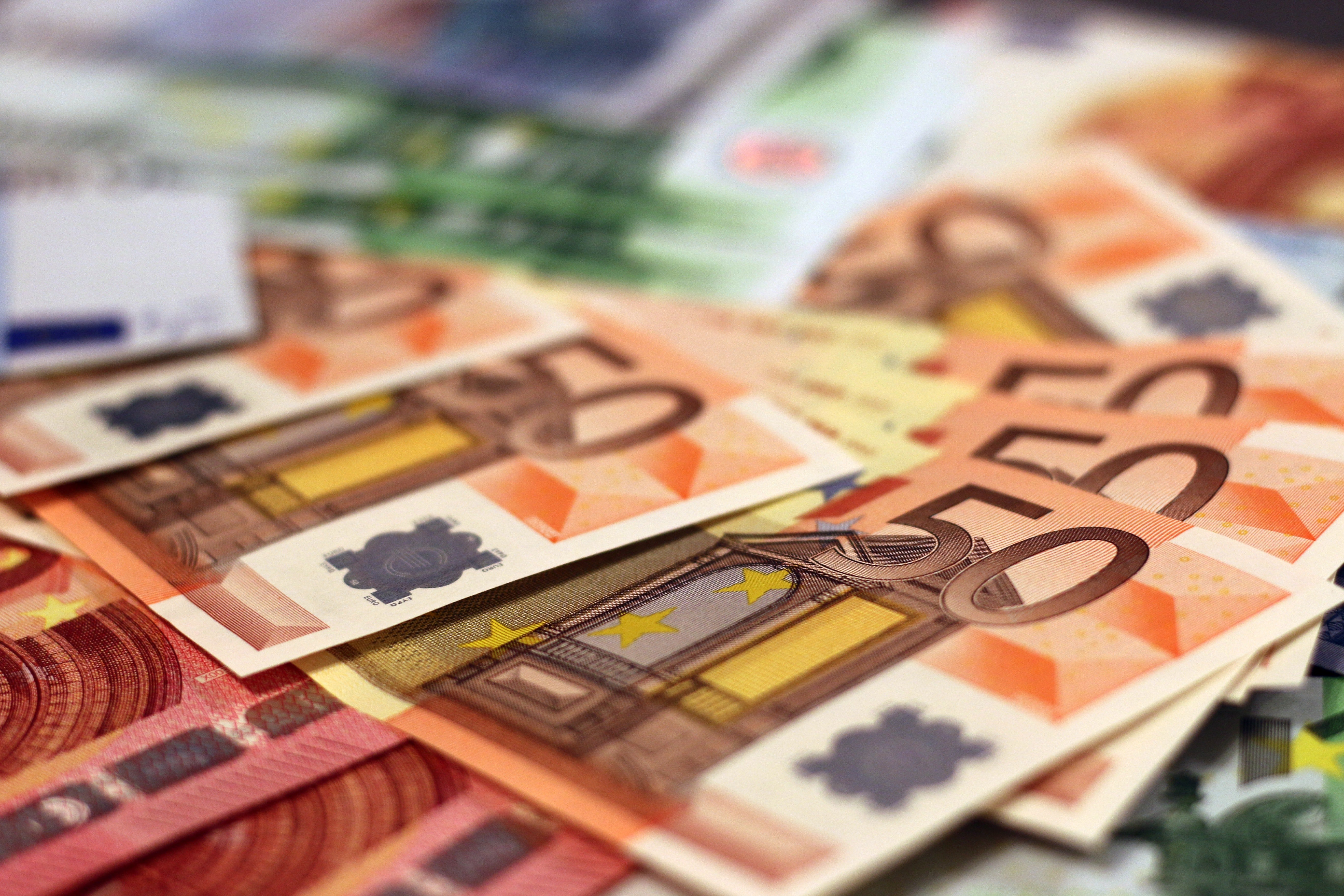﻿ How Is The Value Of A PiP Determined? - FXCM AustraliaHow Is The Value Of A PiP Determined?

To understand how much can be made on a given trade, a trader needs to calculate how much a pip will be worth for a given currency pair. Calculating the final value that a change in pips will be worth depends on the size of currency lots being traded.

Currencies are customarily traded in standard lots, mini lots and micro lots. Standard lots are the largest trading blocs, measured in 100,000 units of a given currency. A standard lot of British pounds, for example, would be £100,000. A mini-lot is equal to 10,000 units of a currency, so one mini-lot of euros would be €10,000. A micro lot is equal to 1,000 units of a currency, so one micro-lot of USD would be US\$1,000.

From One Pip To One Lot

To calculate the value of the individual pip, divide one pip (or 0.0001) by the exchange rate in effect. In the example of the currency pair USD/CHF, if the exchange rate is 1.0451 francs per dollar, then the value of one pip is 0.0001/1.0451 = 0.000095684. The dollar value of the trade can then be determined by multiplying the value of one pip times the lot size traded.

Here are some examples of the different lot sizes:

• If it is a standard lot of 100,000, then the value of a movement of one pip will result in an amount of 0.000095684 x 100,000 = US\$9.57
• For a mini lot of 10,000, the value of the movement of on pip will result in an amount of 0.000095684 x 10,000 = US\$0.96
• For a micro lot of 1,000, the value of the movement of one pip will result in an amount of 0.000095684 x 1,000 = US\$0.09

Trading in the Japanese currency, the yen, will be slightly different, as the pip for the yen is quoted to two decimal points rather than four.

For the currency pair USD/JPY, if the exchange rate is 65.00 yen per dollar, then the value of one pip is 0.01/65.00 = 0.0001538. Here's how that would continue to break down:

• For a standard lot of 100,000, the movement of one pip will result in an amount of 0.0001538 x 100,000 = US\$15.38.
• The calculation for a mini lot of 10,000 would result in amount of an amount of 0.0001538 x 10,000 = US\$1.54
• The calculation for a micro lot would result in amount of 0.0001538 x 1,000 = US\$0.15.

Margins And Leverage

Pip movements can generate even more return or even more losses if a trader has access to leverage. Leverage is a double-edged sword as it can significantly increase losses as well as profits. Leverage is a when a trader multiplies the amount traded by taking a short-term funding allowance from the brokerage. This is done when the trader opens a margin account by making a small good faith security deposit against possible losses.

Some brokerages will allow leverage of up to 50 to 1 with a margin deposit equal to 20% of the position taken. Thus, with a deposit of as little as US\$250, an investor can trade up to US\$100,000. A ten pip move that loses or yields US\$50 on a given contract could then lose or generate ten times as much using leverage.

Leveraged trades, however, are subject to additional risk. If a trade generates a loss that is greater than the investor has in their account, then the trader can incur a negative (debit) balance (and/or a margin call).